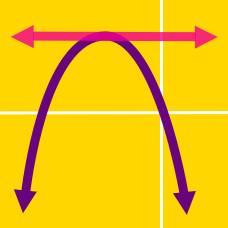Algebra

Completing the Square - Problem Solving

Consider two functions \begin{aligned} f(x) &= -4x^2 + 14 x + 4 \\ g(x) &= -7 x^2 + 8 x + 3. \end{aligned} Let $a$ be the maximum value of $f(x) + g(x) ,$ and $b$ the value of $x$ at that moment. Then what is the value of $a+b?$

For real numbers $x$ and $y$, the polynomial $x^2+2y^2+2xy-8x-10y+30$ has a minimum value $m$ at $x=a$ and $y=b$. What is the value of $abm$?

Let $f(x)= 5 x + 60$ and $g(x)= 4 x + 80.$ If $a$ is the minimum value of $f(x) \times g(x)$ and $b$ is the value of $x$ at that moment, what is the value of $b-a?$

Consider two points $A=(2,5)$ and $B=(4,4)$, and a third point $P=(a, 0)$ on the $x$-axis. If the minimum value of $\overline{PA}^2+\overline{PB}^2$ is $c$, what is $a+c$?

Consider the parabola $y = x^2 + k^4 + p^4 -2k^2 x -2p^2x + 2k^2p^2 + 36,$ where $k$ and $p$ are parameters. If the vertex of the parabola lies on the line $y = \frac{ 1 }{25} x,$ what is the value of $k^2 + p^2?$

×